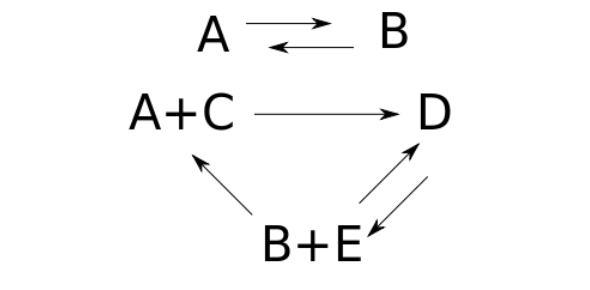# Reversible Reactions Quiz Questions And Answers

7 Questions | Attempts: 1566
ShareSettingsA reversible reaction is a chemical reaction where the reactants form products that, in turn, react together to give the reactants back. Are you preparing for exams on the subject? This quiz will help you do that.

• 1.
2NH3(g) <------>  N2(g) + 3H2(g) ;  Heat of reaction = +92 kJThe amount of ammonia in the equilibrium mixture can be made larger by
• A.

Changing the catalyst.

• B.

Lowering the temperature.

• C.

Lowering the pressure.

• D.

Removing some of the hydrogen.

• 2.
When a reversible reaction reaches dynamic equilibrium at constant temperature,
• A.

The reaction stops.

• B.

Equal amounts of reactants and products are present.

• C.

The ratio of the product concentration to the reactant concentration is constant.

• D.

The products of the forward reaction stop reacting altogether.

• 3.
The equation shows the equilibrium between A, B and C.A (g) + 2 B (g)  <--->  2 C (g)       ΔH = +150 kJHow can the amount of B in the equilibrium mixture be increased?
• A.

• B.

Lowering the temperature

• C.

Removing some of C

• D.

Increasing the pressure

• 4.
In which reaction will be amount of product be increased by decreasing the pressure?
• A.

2NO (g) + O2(aq) 2NO2 (g)

• B.

N2 (g) + 3H2 (g) 2NH3 (g)

• C.

2NO2 (g) N2O4 (g)

• D.

CH4 (g) + H2O (g) CO (g) + 3H2 (g)

• 5.
The equation show the equilibrium between silver ions and iron(II) ions.Ag+(aq) + Fe2+(aq) <----> Ag(s) + Fe3+(aq) Which one of the following statements is not true for the above equilibrium?
• A.

At equilibrium, the rate of the forward reaction is equal to the rate of the backward reaction.

• B.

The concentration of all reactants and products are independent of the external pressure.

• C.

Addition of dilute hydrochloric acid to precipitate silver chloride would cause the equilibrium to shift to the left.

• D.

The yield of silver metal could be significantly increased by adding a catalyst.

• 6.
On 6 & 7.In the Haber Process, N2(g) + 3H2(g) <------>  2NH3(g) The following graph shows the effect of temperature and pressure on the yield.Which of the following options does not explain why the yield increases when temperature decreases?
• A.

The system responds in a way so as to counteract the decreased temperature.

• B.

The system favours the forward reaction which is exothermic.

• C.

The system favours the forward reaction which is endothermic.

• D.

The system responds in a way in accordance with Le Chatelier's principle, replacing the heat lost by raising the temperature.

• 7.
Which of the following options does not explain why the yield increases when pressure is increased?
• A.

The volume of gas molecules decreases in the forward reaction.

• B.

The number of moles of gas molecules increases in the forward reaction.

• C.

An increase in pressure due to decreasing volume causes the reaction to shift to the side with the fewer moles of gas.

• D.

The position of equilibrium shifts to the right to favour the forward reaction.

## Related TopicsBack to top
×

Wait!
Here's an interesting quiz for you.